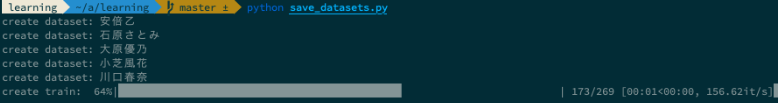# Introduction

――The total size of the learning image, test image, and padded image this time is about `1.4GB`. ――It will take some time to load these images when the learning program is implemented. —— Also, when you run a learning program in another environment, transfer time will occur. ――In addition, it takes time to resize the image and convert from color to gray. ――By creating a dataset that has been resized and converted to gray in advance, we were able to make it about `50MB`. --The complete source is here.

# Library

--Like last time, I'm using `Numpy`` Pillow`.

# Setting

--The following settings have been added. --The dataset created this time is saved in `DATASETS_PATH`. --ʻIMG_ROWS` ʻIMG_COLS` is a resizing of the image size. This time, we will resize it to the size of `28 x 28`. --The image size is also referred to in the training model of the post-process.

#### `config.py`

``````
DATASETS_PATH = os.path.join(DATA_PATH, 'datasets')

IMG_ROWS, IMG_COLS = 28, 28
``````

# Creating a file list

--Create a file list of training images, test images, and padded images. --query is given `CLASSES` in sequence. --ʻAugment`The argument is a flag for the availability of padded images. --Last time, the padded image was created for each`query`` 6000`. If it is not enough, it is an error.

``````def make_filesets(augment):
"""Creating a file set."""

filesets = {'train': dict(), 'test': dict(), 'augment': dict()}

for query in CLASSES:

train_path = os.path.join(TRAIN_PATH, query)
test_path = os.path.join(TEST_PATH, query)
augment_path = os.path.join(AUGMENT_PATH, query)

if not os.path.isdir(train_path):
print('no train path: {}'.format(train_path))
return None
if not os.path.isdir(test_path):
print('no test path: {}'.format(test_path))
return None
if not os.path.isdir(augment_path):
print('no augment path: {}'.format(augment_path))
return None

train_files = glob.glob(os.path.join(train_path, '*.jpeg'))
train_files.sort()
filesets['train'][query] = train_files

test_files = glob.glob(os.path.join(test_path, '*.jpeg'))
test_files.sort()
filesets['test'][query] = test_files

augment_files = glob.glob(os.path.join(augment_path, '*.jpeg'))
random.shuffle(augment_files)
filesets['augment'][query] = augment_files

if augment and len(augment_files) < AUGMENT_NUM:
print('less augment num: {}, path: {}'.format(len(augment_files), augment_path))
return None

return filesets
``````

--Processes the image based on the full path of the file. --It will be resized according to the configuration file. --Originally, in OpenCV Haar Cascades, it was saved without resizing. Resizing in a later process is more convenient to try in various sizes. ―― `LANCZOS` takes time, but it resizes with good quality. The default is `NEAREST`. Speed is prioritized over quality. --Reference: https://pillow.readthedocs.io/en/4.0.x/handbook/concepts.html#filters --Then, convert to grayscale and then to ʻuint8`.

``````def read_image(filename):

image = Image.open(filename)
image = image.resize((IMG_ROWS, IMG_COLS), Image.LANCZOS)
image = image.convert('L')
image = np.array(image, dtype=np.uint8)

return image
``````

# Creating a dataset

--Prepare an array of training images, training labels, test images, and test labels.

``````def make_datasets(augment, filesets):
"""Creating a dataset."""

train_images = []
train_labels = []
test_images = []
test_labels = []
``````

--query is given `CLASSES` in sequence. --Num is given a sequential label. ――For example, at the beginning of `CLASSES`, the` Abe Oto` label is like `0`. --Determine whether to use the inflated image with ʻaugment`. If you want to use it, set only the number described in ʻAUGMENT_NUM` to` train_files`. --The `tqdm` is also used to read each image. The processing progress is displayed, which is easy to understand. --Give `read_image` the file path of the image to read the resized and grayscaled image. --At the same time, give a label.

``````    for num, query in enumerate(CLASSES):
print('create dataset: {}'.format(query))

if augment:
train_files = filesets['augment'][query][:AUGMENT_NUM]
else:
train_files = filesets['train'][query]
test_files = filesets['test'][query]

for train_file in tqdm.tqdm(train_files, desc='create train', leave=False):
train_labels.append(num)
for test_file in tqdm.tqdm(test_files, desc='create test', leave=False):
test_labels.append(num)
``````

--Collect training images, training labels, test images, and test labels as a data set. --`DATASET_PATH`` CLASSES` ʻIMG_ROWS` ʻIMG_COLS` Decide the file name of the dataset based on whether or not the padded image is used.

``````    datasets = ((np.array(train_images), (np.array(train_labels))), (np.array(test_images), (np.array(test_labels))))

datasets_path = os.path.join(DATASETS_PATH, ','.join(CLASSES))
os.makedirs(datasets_path, exist_ok=True)
train_num = AUGMENT_NUM if augment else 0
datasets_file = os.path.join(datasets_path, '{}x{}-{}.pickle'.format(IMG_ROWS, IMG_COLS, train_num))
with open(datasets_file, 'wb') as fout:
pickle.dump(datasets, fout)
print('save datasets: {}'.format(datasets_file))
``````――The use of inflated images is switched by the following options.

``````\$ python save_datasets.py

\$ python save_datasets.py --augment
``````

--The pickle data set is as follows. --In the original case, a pickle file of `train`` test` totaling about `148MB` to` 3.2MB` --For padded images, pickle files from ʻaugment`` test`totaling about`1433MB` to` 46MB`

``````\$ du -d1 -h .
115M	./train
33M	./test
51M	./datasets
1.4G	./augment

\$ ls
3.2M 12 15 23:22 28x28-0.pickle
46M 12 15 22:24 28x28-6000.pickle
``````

# in conclusion

――We have created a data set that resized and grayscaled the image data so that it can be easily used from the learning program. ――You can create various data sets by changing the number of inflated images and multiple sizes, and use them while switching by file name. ――Next time, I plan to create a part that reads the dataset from the learning program.